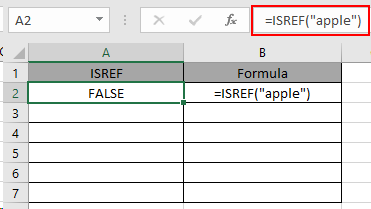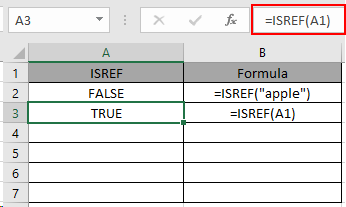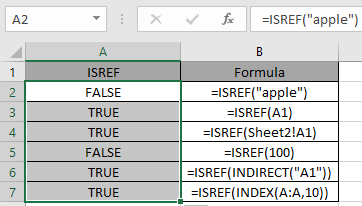# How to use the ISREF Function in Excel

ISREF function returns TRUE if the value is a reference and it returns FALSE if not. Reference to the function is the value referred indirectly to the values. Reference to the function can be given in many forms. See the example below used with the function.
ISREF function checks the value cell for a reference value and returns TRUE if exist or else FALSE.

Syntax:

=ISREF ( value )

value : value to the function can be given as directly or using cell reference

Now let’s understand the function more via using it in an example

Below in one column is the output of the ISREF function and in the second column there are the formulas to get the corresponding resultHere we have a list of value in Column A & we need to check the cells for the reference values.

Text input returns FALSE as the input is not a reference.Here the function returns TRUE as the A1 is used as cell reference to the function.You can observe the output from the above snapshots

As you can see, the function returns the logic values either TRUE or FALSE. Below are some of the observational results.

Note:

1. When range of cells given as argument, the function only checks the first cell of the array as returns TRUE or FALSE based only on the first cell.
2. The function returns TRUE when the reference is inside the inner functions.
3. The function returns TRUE when it is used with INDIRECT function as INDIRECT function itself is reference function.

Hope you understood how to use ISREF function in Excel 2016. Find more articles on Excel ISREF function here. Please share your query below in the comment box. We will assist you.

Terms and Conditions of use

The applications/code on this site are distributed as is and without warranties or liability. In no event shall the owner of the copyrights, or the authors of the applications/code be liable for any loss of profit, any problems or any damage resulting from the use or evaluation of the applications/code.